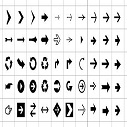# hackerrank Cut the sticks problem

You are given a number of sticks of varying lengths. You will iteratively cut the sticks into smaller sticks, discarding the shortest pieces until there are none left. At each iteration you will determine the length of the shortest stick remaining, cut that length from each of the longer sticks and then discard all the pieces of that shortest length. When all the remaining sticks are the same length, they cannot be shortened so discard them.

Given the lengths of sticks, print the number of sticks that are left before each iteration until there are none left.

For example, there are sticks of lengths . The shortest stick length is , so we cut that length from the longer two and discard the pieces of length . Now our lengths are . Again, the shortest stick is of length , so we cut that amount from the longer stick and discard those pieces. There is only one stick left, , so we discard that stick. Our lengths are .

Function Description

Complete the cutTheSticks function in the editor below. It should return an array of integers representing the number of sticks before each cut operation is performed.

cutTheSticks has the following parameter(s):

• arr: an array of integers representing the length of each stick

Input Format

The first line contains a single integer , the size of .
The next line contains space-separated integers, each an where each value represents the length of the stick.

Output Format

For each operation, print the number of sticks that are present before the operation on separate lines.

def cutTheSticks(arr):
result = []
while arr:
shortest = min(arr)
result.append(len(arr))
arr = list(filter(lambda x : x - shortest, arr))
return resultAkd on Jul 14, 2020 at 12:07 am

#### Post Comment

##### WEB TECHNOLOGY##### TypeScript Programmning Language##### New HTML5 APIs##### HTML Arrow -> Range: Decimal 8592-8703. Hex 2190-21FF.##### How To Override One CSS Class With Another×